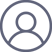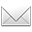李毅伟2019-4-26 09:25············* * *

Theorem 1.5. There is a homeomorphism of topological spaces |(A¹K )ᵃᵈ| lim<|(A¹K)ᵃᵈ| (TT).
---- 存在拓扑空间同构/同胚: |(A¹K )ᵃᵈ| lim<|(A¹K)ᵃᵈ| (TT).
.
Note that both sides of this isomorphism can be regarded as locally ringed topological spaces.
---- 此同构的两端都可看作局部环拓扑空间.
.
It is natural to ask whether one can compare the structure sheaves on both sides.
---- 自然要问，可否比较两端的结构 sheaves.

（我们的教授大多武断，或不允许提出此等问题，也就不会有下文了）
.
There is the obvious obstacle that the left-hand side has a sheaf of characteristic p rings, wheeas the right-hand side has a sheaf of characteristic 0 rings.
---- 明显的障碍是，左端有一沓特征p环，右端则有一沓特征0环.
.
Fontaine's functors make it possible to translate between the two worlds.
---- Fontaine 函子使得这两个世界之间的翻译成为可能.
.
There is the following result.
---- 有如下结果.
.

*

1. K lim<A¹K (TT).
2. [K] = lim<[K] (x x).
3. X(K)~Xᵃᵈ(K)~|Xᵃᵈ|.

---- K°/p  K°/p.(para.3a)
---- K = lim<K, x x^p.(para.3b)
----  (x)d --> (x#)d
↑  分裂域   ↓
[K] ~>  [K]c
： x:=akn.(para.3c)
---- ndv(1)~K~(Φ)=K/p.
---- K(p)~Fontaine~K.
---- {K} {K}. (Th1.3)